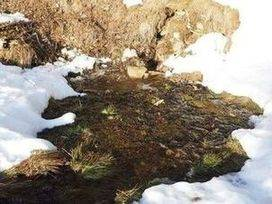# 4 Top problems of Water Seepage in soilsWater seepage is the movement of groundwater through the soil under a hydraulic gradient when there is a difference in the hydraulic head at the two points.

## 4 Top problems of water seepage in soils

The following 4 top problems of water seepage in soils :

1. In the foundation or earth dam, there will be loss of storage water.
2. Due to consolidated effect, there will be a settlement of structures that are constructed on compressible soils.
3. Soils slopes will be instable.
4. There will be forces on hydraulic structures due to the force exerted by the percolating water.

For studying seepage forces Laplace equation is used.

The solution of two Laplace equations for the flow functions and potential functions takes the form of two sets of orthogonal curves.

## Streamlines or Flowlines

Under laminar flow, the path followed by percolating water through a saturated soil mass is called flow line.

### Equipotential line

The equipotential line is the line joining the points of equal hydraulic potentials.

The joining points of this line have an equal total head.

Along an equipotential line, if piezometers were inserted into the soil at different points, water would rise to the same elevation in all these piezometers.

The pressure heads, elevation heads are different but total head is constant along the equipotential line. This line represents lines of the equal head.

The loss of heads caused by water crossing two adjacent equipotential lines is called the potential drop.

### Flow channel

Between two adjacent flow lines, a portion of the flow net is called a flow channel.

### Flow Field

Between two adjacent equipotential lines, the located section of a flow channel is called flow field.

### Flow Net

A system of flow lines and equipotential lines forms a network called flow net.

It is the pictorial representation of the pattern of flow and the dissipation of hydraulic head causing the flow in soil.

#### Properties of Flow net:

The following are the properties of flow net:

1. Equipotential lines and flow lines are perpendicular to each other.
2. Between any two adjacent equipotential lines, the potential drop is the same.
3. The water quantity flowing through adjacent flow lines is the same.
4. In small dimensional square figures, the flow velocity is more so as to keep discharge same.

#### Water Seepage Quantity

The total discharge through the complete flow net per unit length can be calculated by:

q = HK (Nr/Nd).

Where, Nf=Number of flow channels.

Nd=Number of potential drops, H=Tota head loss.

The ratio of Nf/Nd is called the shape factor of the flow net.

### Amount of seepage pressure

The amount of seepage pressure is given by the formula:

Seepage pressure = rw(H-Nd(h2-h1)

This pressure of seepage acts on the direction of flow.

### Amount of Uplift pressure

The amount of uplift pressure is given by:

Uplift pressure = hw.rw

At the downstream end of the flow, there will be a maximum hydraulic gradient known as the exit gradient.

It is also known as the hydraulic gradient where the percolating water leaves the mass of soil and emerges into free water.

This exit gradient is given by, ie= head loss(h2-h1)/L

where L= Lenght of the smallest square in the field.

#### Phreatic line:

This phreatic line is also called water seepage line or top flow line.

Along this line, only atmospheric pressure exits and pressure head is zero and this line attains parabolic shape.

Seepage through earth dam is calculated using the kozey’s solution. Discharge through the body of a dam is given by q = KS.

where S= focal distance, i.e distance between focus and direction of the parabolic shape phreatic line.

## Water Seepage in Anisotropic soils:

For the soils having different permeabilities, flow lines get deflected at the interface. The equipotential lines also get deflected at the interface of the soils.

## Piping failure due to water seepage

Due to the high value of the hydraulic gradient, there will be instability at the end face of percolating soil mass, the soil grains are dislodged and are eroded.

At the exit of such erosions gradually a pipe-shaped discharge channel will form.

The hydraulic gradient and the width of this pipe-shaped channel will increase with time and will lead to a failure of the structure which is constructed on the soil. Such type of failure is called piping failure.

## Piping failures due to water seepage

Drain materials or filters are used for preventing piping failures due to water seepage.

The following requirements should satisfy by drain materials or filters:

1. The material gradation should be such that it should allow rapid drainage without developing the seepage forces.
2. The filter material gradation should be such that it should allow rapid drainage without developing the seepage forces.
3. The filter gradation material should be capable of preventing the migration of adjacent particles through pores by forming the small size pores.
4. The material should add some self-weight to the structure.

```2 shallow foundations | Settlements